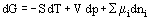# CHEMICAL POTENTIAL

The chemical potential of the i-component of a thermodynamic system in a given phase is a thermodynamic state function. It defines changes of the Gibbs energy and other thermodynamic potentials when the number of particles of a corresponding component is changed. Chemical potential of the ith-component of the system is the derivative of any thermodynamic potential divided by the quantity (or number of molecules) of this component when the values of the other thermodynamic variables, given a thermodynamic potential, are constant, e.g., μi = (G/ni)T,p,nj, where G is the Gibbs energy of the phase; ni, the number of moles of the i-component of the phase; T, the absolute temperature; p, pressure, and nj, the number of moles of all other components (j = i). It is obvious that chemical potential (μi) is a partial molar Gibbs energy. The sum ∑μidni, which enters into the expression for the total differential of all thermodynamic potentials, has been called the fundamental Gibbs equation, e.g.,:where S is the entropy and V the volume.
The conditions of thermodynamic equilibrium can be determined in the simplest way by using chemical potentials. If the sum of the products of chemical potentials and stoichiometric coefficients for all reagents is equal to zero, then this equality is a condition of chemical equilibrium. It is assumed that the values of stoichiometric coefficients of the reaction products are negative. The condition of phase equilibrium is the equality of chemical potentials for each component in all phases. If an equilibrium system is homogeneous, the chemical potential of each component at all points of the system is identical.
Chemical potential is expressed in energy units per unit of the substance mass (Joule/kg) or per mole of the substance (Joule/mole) or per molecule of the substance. The term "chemical potential" was coined by J. Gibbs (1875), a prominent American physicist.